# FLuent doesn't take into account the udf's code

Hello everyone

I would like to simulate a fluid flow over a flat plate under a body force by using UDF code.

My problem is that the result is like a model without body force.

Below, you find my approach.

1. Interpreted to UDF code.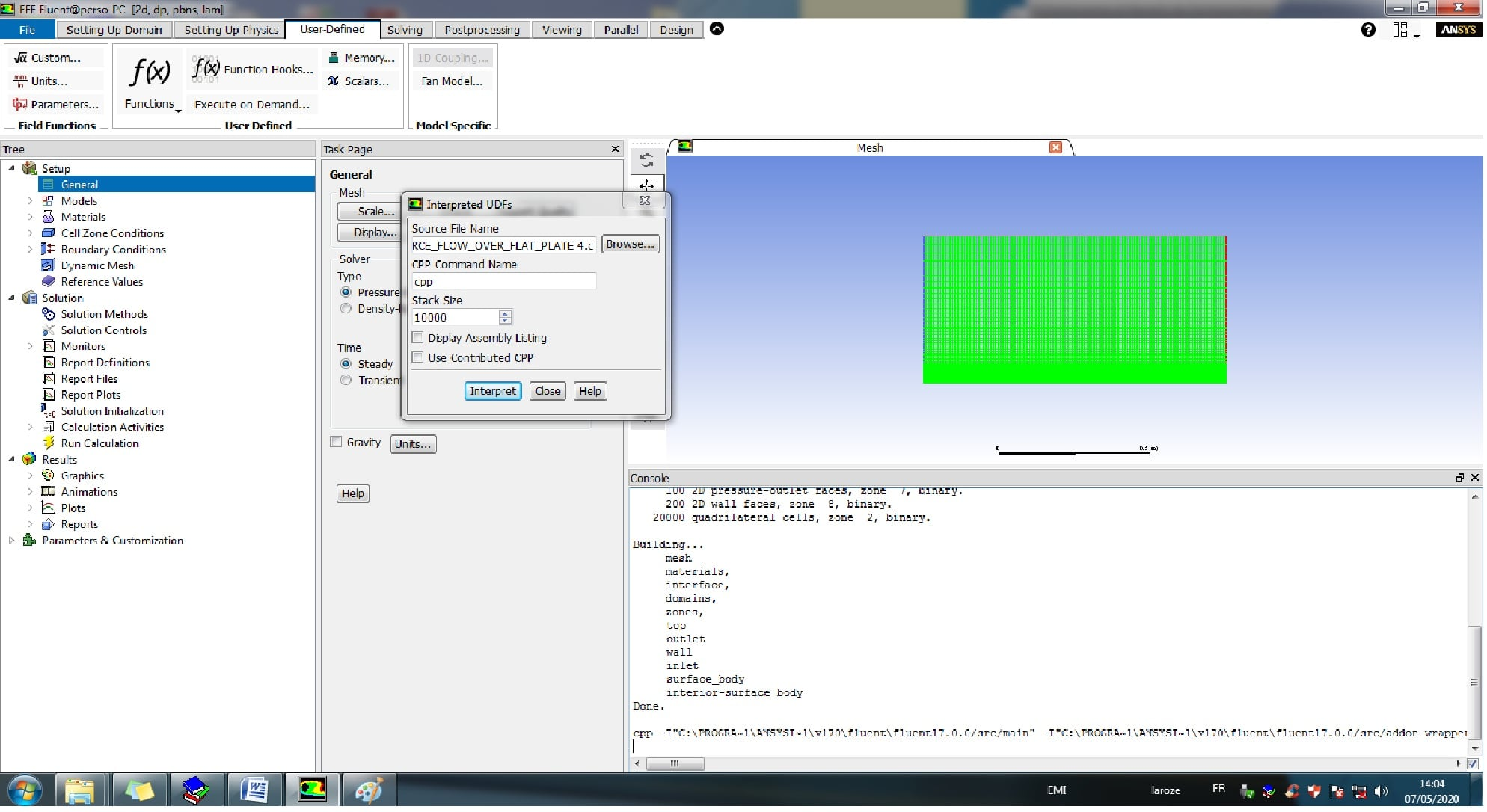2. Hooking

2.1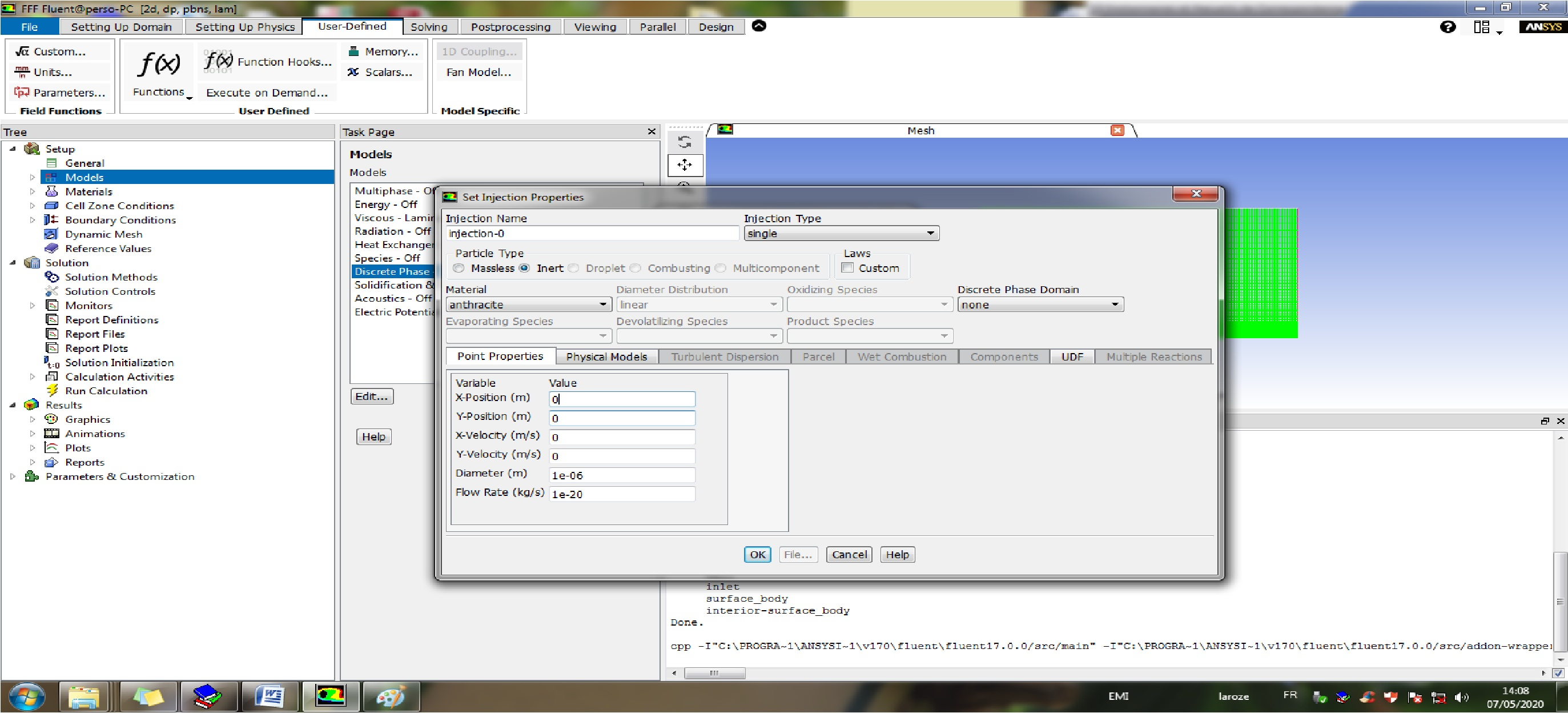2.2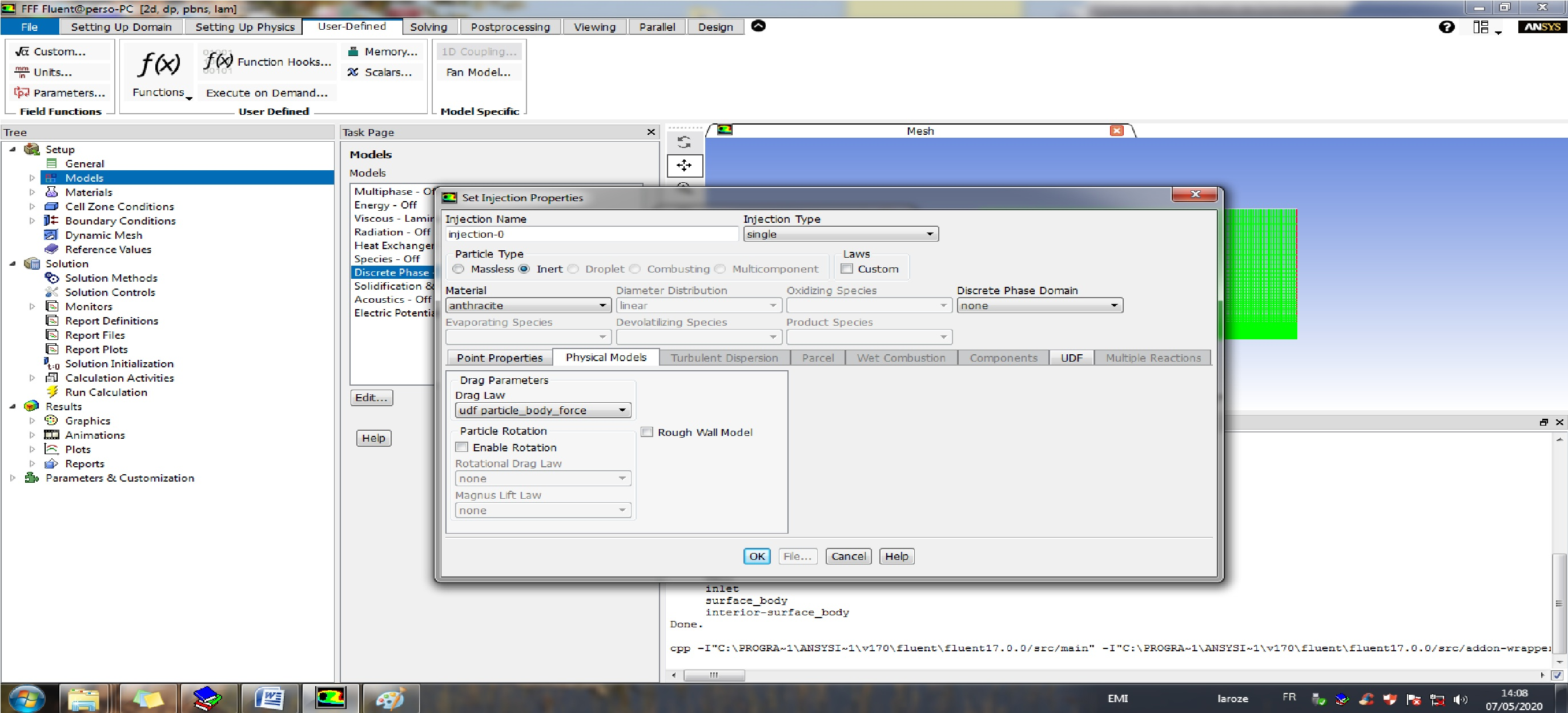2.3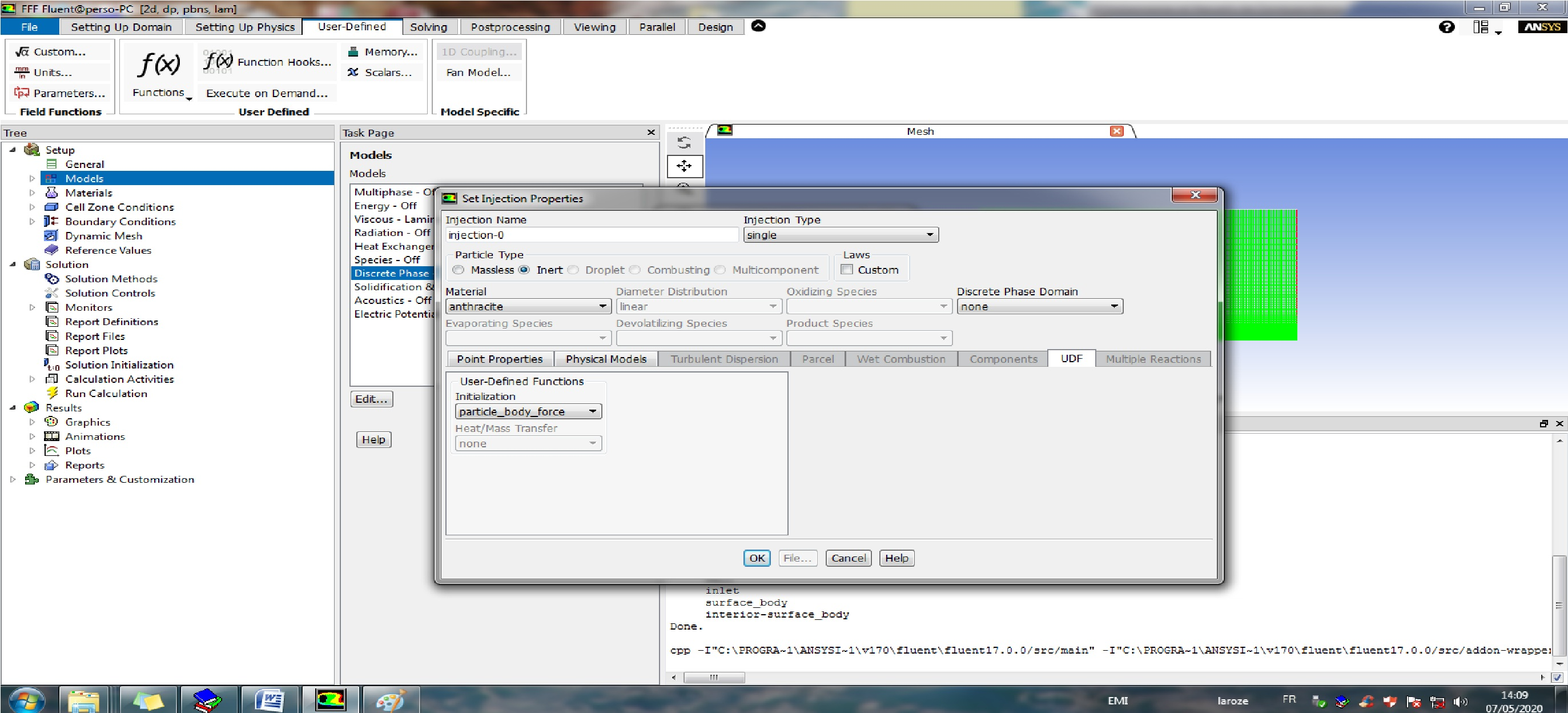2.4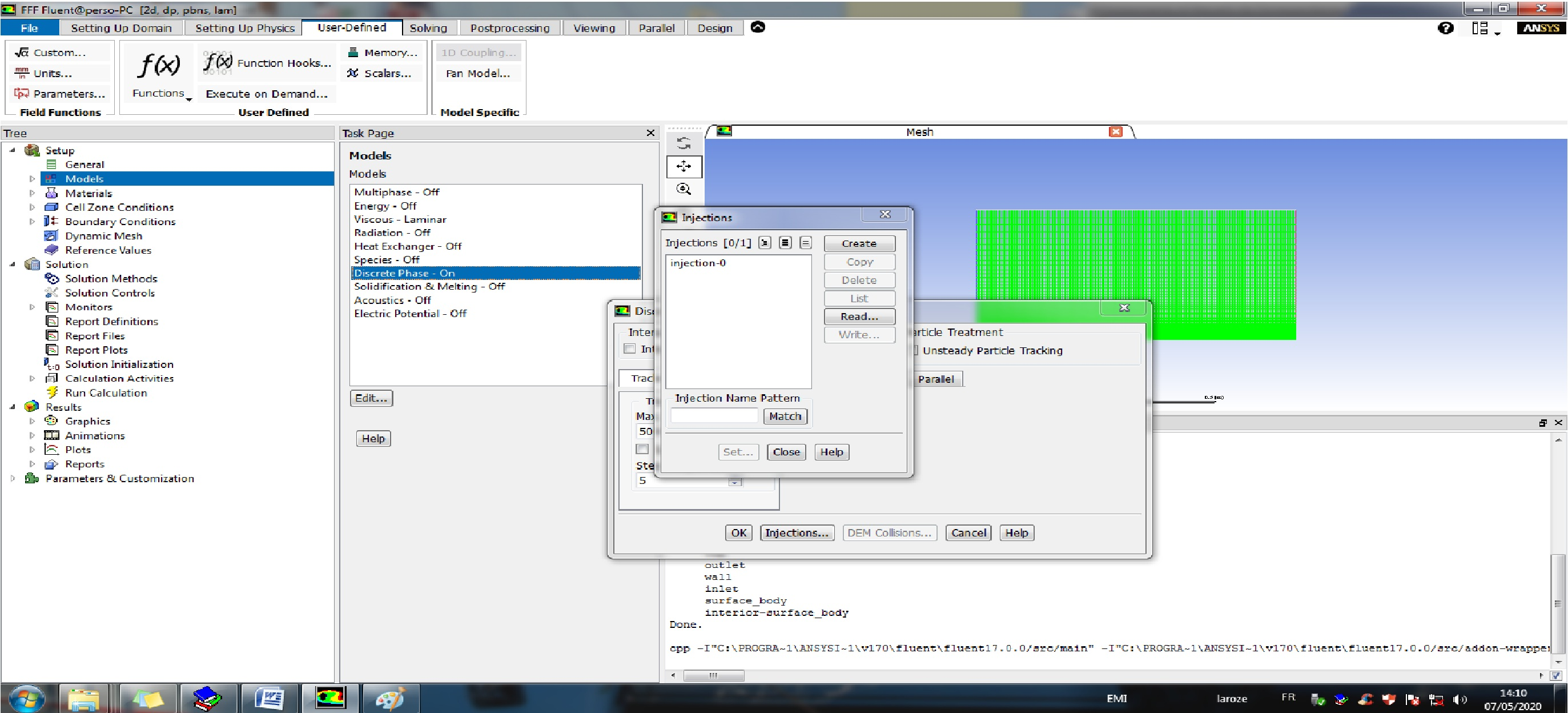2.5 My problem is the lack of ::libudf ( according to the UDF manual)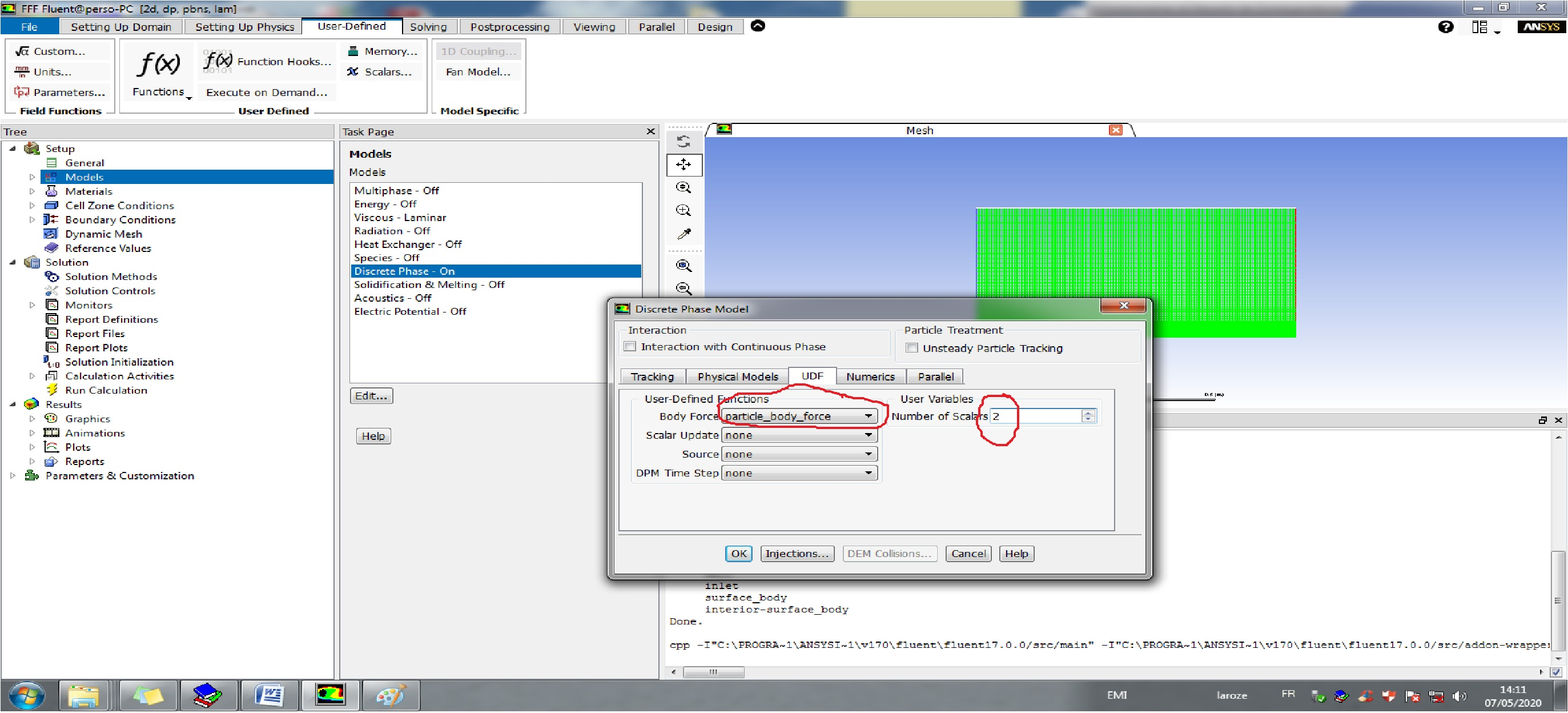3. Models===> Discret phase on

4. cell Zone Condition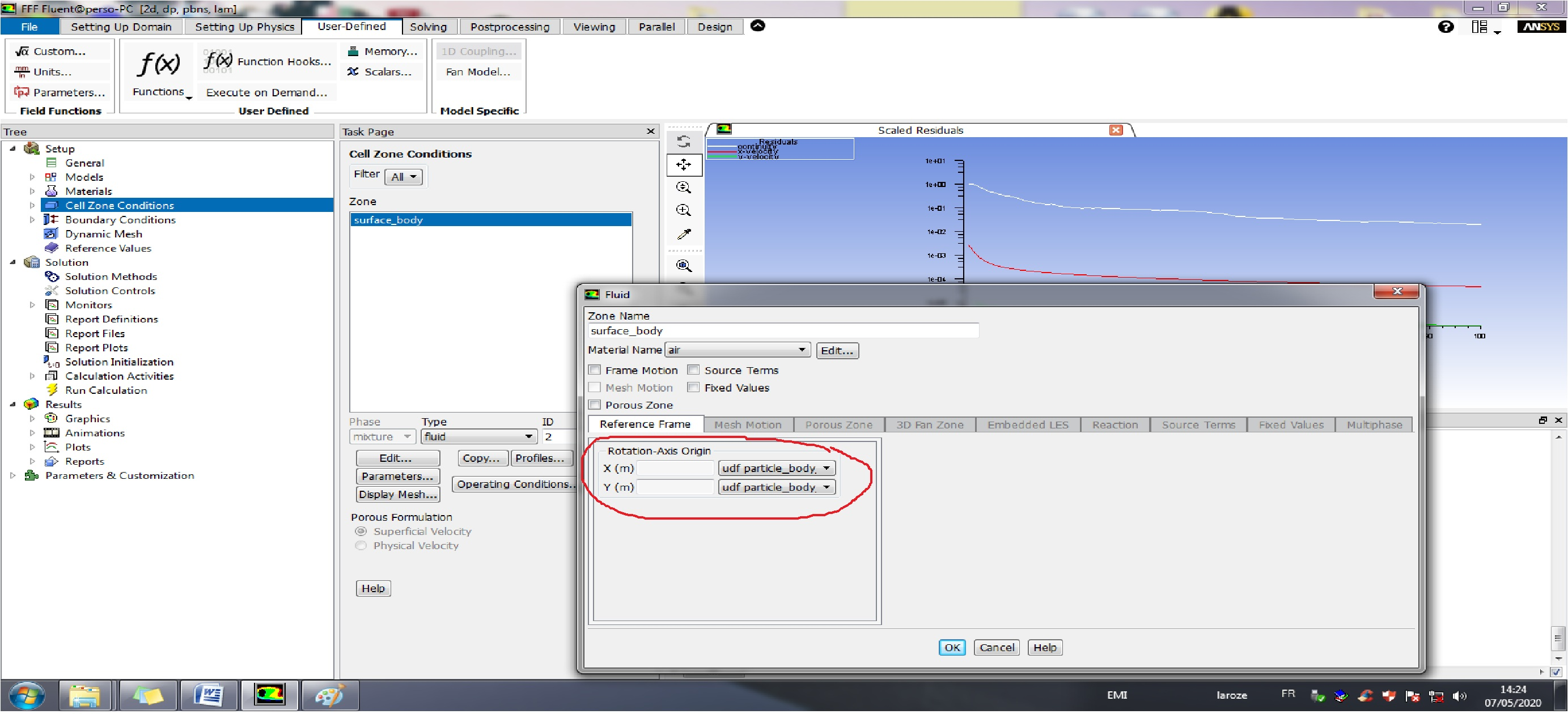5. Boundary Conditions :

5.1 Inlet velocity: have I to do 1 m/s or udf ?

In fact, In UDF code I have just coded the body force without inlet velocity.
UDF code: x=0  bforce=0

x!=0 bforce (i==0)= F(x,y)

bforce (i==1)=G(x,y)

Can I use 02 macros in one udf code ? : Body_Force and DPM_BC (Velocity inlet)

6. Solution Methodes

>>> Pressure-velocity coupling : simple   (Without change)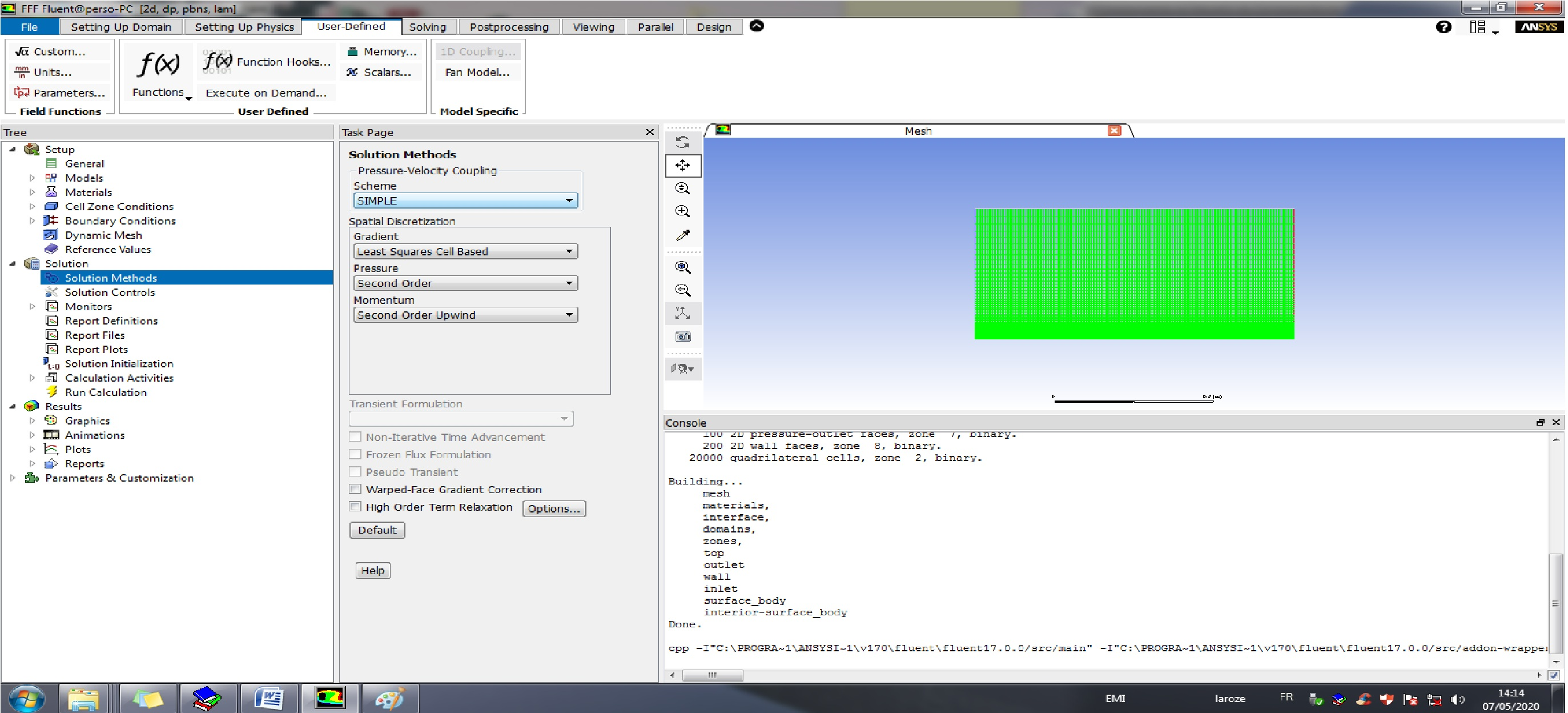7. Solution Controls (without change)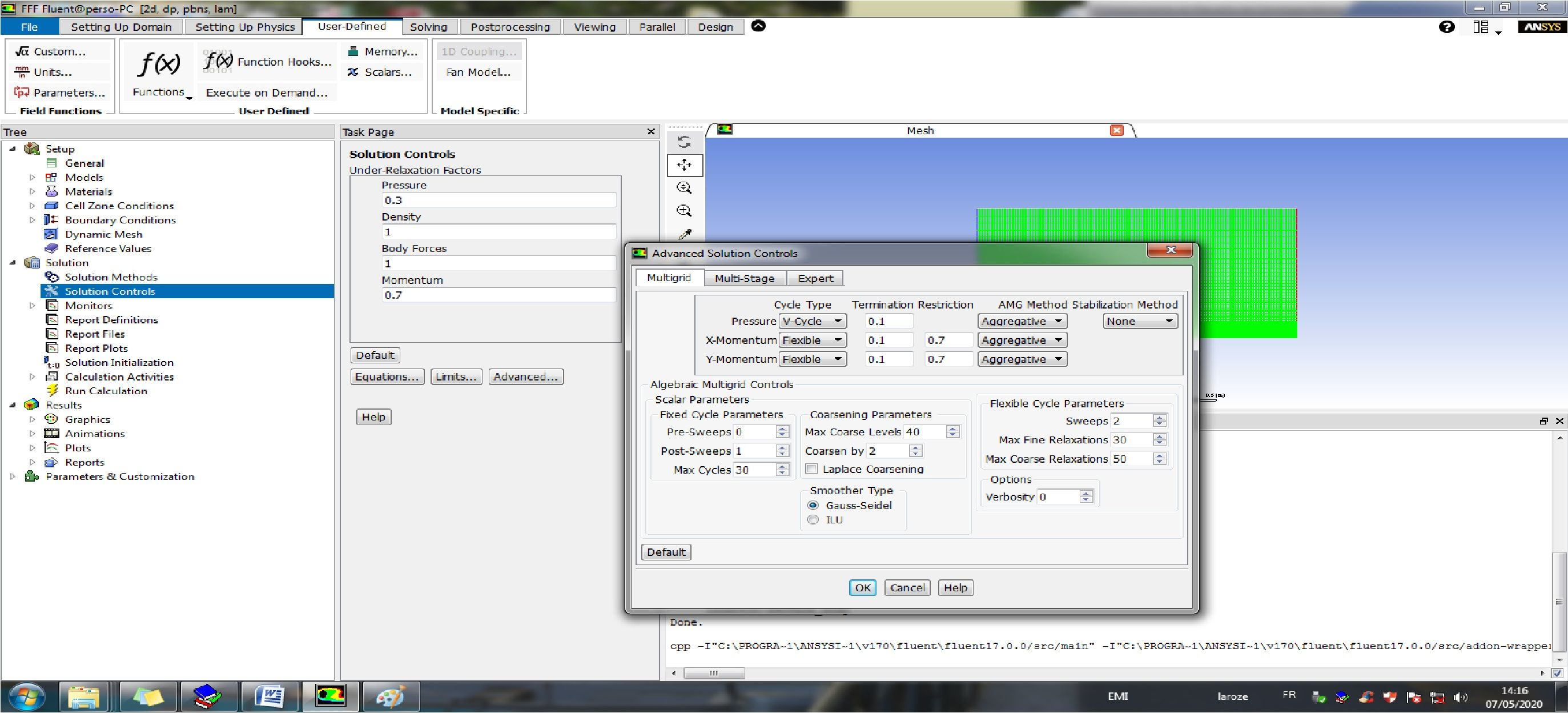7. Solution Initialization

>> STANDARD INITIALIZATION

>>> COMPUTE FORM: ALL-ZONES

>>>> x-velocity 1 m/s

>>> Initialize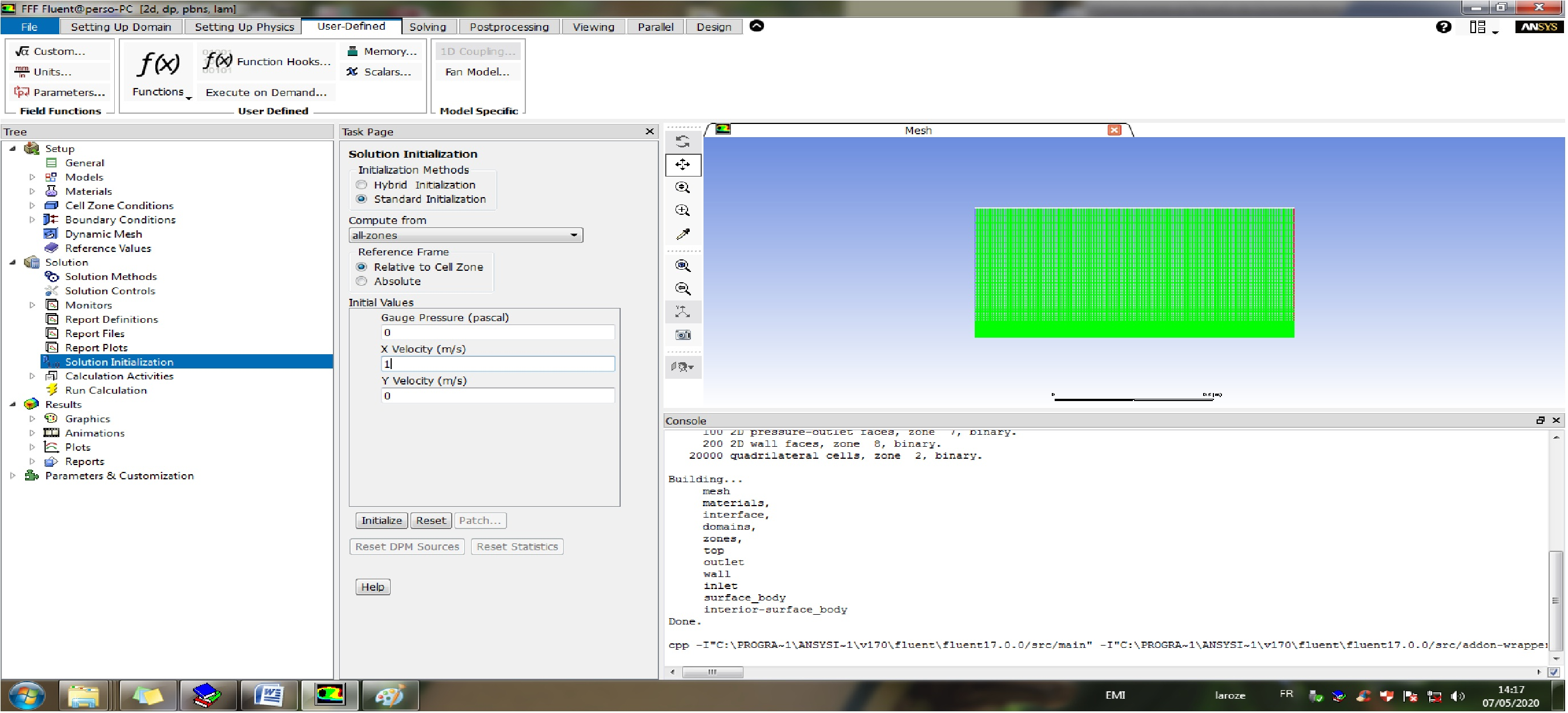8. Run calculation : The programm doesn't take into account the udf code .(The result is same to model without udf)

thanks you

• edited May 5
which approach?
•

ANSYSCOMP posted this 3 days ago - Last edited yesterday

Hello everyone

I would like to simulate a fluid flow over a flat plate under a body force by using UDF code.

My problem is that the result is like a model without body force.

Below, you find my approach.

1. Interpreted to UDF code.2. Hooking

2.12.22.32.42.5 My problem is the lack of ::libudf ( according to the UDF manual)3. Models===> Discret phase on

4. cell Zone Condition5. Boundary Conditions :

5.1 Inlet velocity: have I to do 1 m/s or udf ?

In fact, In UDF code I have just coded the body force without inlet velocity.
UDF code: x=0  bforce=0

x!=0 bforce (i==0)= F(x,y)

bforce (i==1)=G(x,y)

Can I use 02 macros in one udf code ? : Body_Force and DPM_BC (Velocity inlet)

6. Solution Methodes

>>> Pressure-velocity coupling : simple   (Without change)7. Solution Controls (without change)7. Solution Initialization

>> STANDARD INITIALIZATION

>>> COMPUTE FORM: ALL-ZONES

>>>> x-velocity 1 m/s

>>> Initialize8. Run calculation : The programm doesn't take into account the udf code .(The result is same to model without udf)

thanks you